# Creating plots in R using ggplot2 - part 6: weighted scatterplots

written in

This is the fifth tutorial in a series on using ggplot2 I am creating with Mauricio Vargas Sepúlveda. In this tutorial we will demonstrate some of the many options the ggplot2 package has for creating and customising weighted scatterplots. These plots are also called ‘balloon plots’ or ‘bubble plots’. We will use R’s airquality dataset in the datasets package. In order to reduce the complexity of these data a little, we will only be looking at the final three months in the dataset (July, August and September).

If you enjoyed this blog post and found it useful, please consider buying our book! It contains chapters detailing how to build and customise all 11 chart types published on the blog, as well as LOWESS charts. The book is also actively maintained (unlike the series on the blog) and contains up-to-date ggplot and tidyverse code, and every purchase really helps us out with keeping up with new content.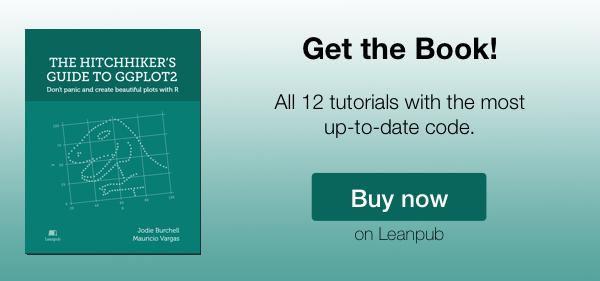The first thing to do is load in the data, as below:

rm(list = ls())
library(datasets)
library(ggplot2)
data(airquality)


We will then trim the data down to the final three months and turn the Month variable into a labelled factor variable. We end up with a new dataset called aq_trim.

aq_trim <- airquality[which(airquality$Month == 7 | airquality$Month == 8 |
airquality$Month == 9), ] aq_trim$Month <- factor(aq_trim\$Month,
labels = c("July", "August", "September"))


In this tutorial, we will work towards creating the weighted scatterplot below. We will take you from a basic scatterplot and explain all the customisations we add to the code step-by-step.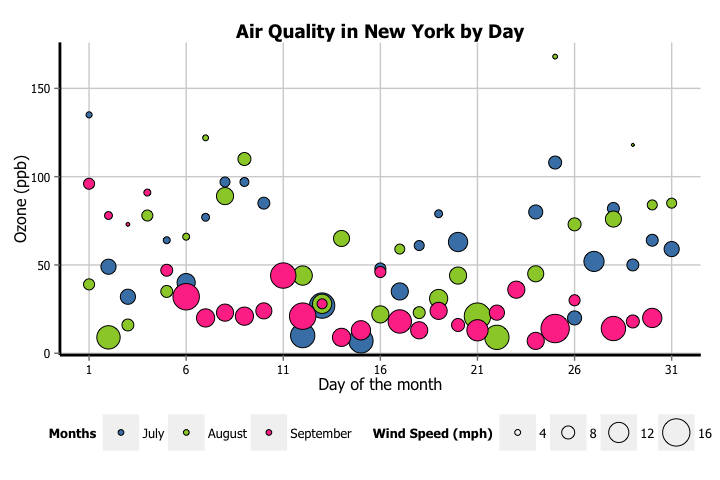### Basic weighted scatterplot

Let’s start really slowly by revisiting how to create a basic scatterplot. In order to initialise this plot we tell ggplot that aq_trim is our data, and specify that our x-axis plots the Day variable and our y-axis plots the Ozone variable. We then instruct ggplot to render this as a scatterplot by adding the geom_point() option.

p6 <- ggplot(aq_trim, aes(x = Day, y = Ozone)) +
geom_point()
p6In order to turn this into a weighted scatterplot, we simply add the size argument to ggplot(aes()). In this case, we want to weight the points by the Wind variable.

p6 <- ggplot(aq_trim, aes(x = Day, y = Ozone, size = Wind)) +
geom_point()
p6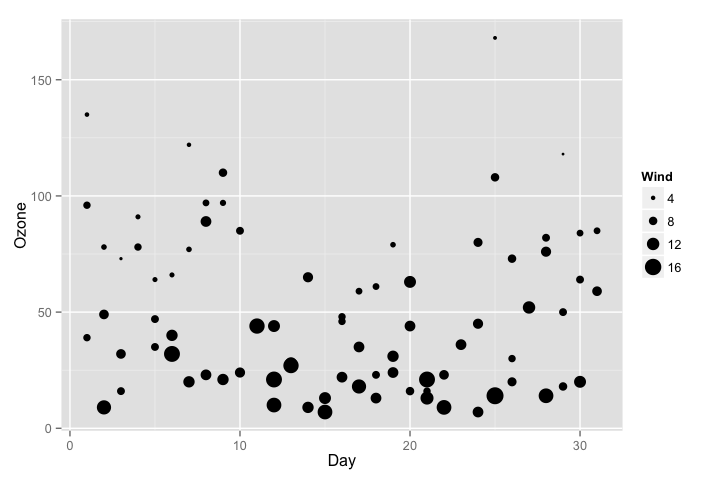You can see we already have an interesting looking pattern, where days with higher wind speed tend to have lower ozone (or in other words, better air quality). Now let’s make it beautiful!

### Changing the shape of the data points

Perhaps we want the data points to be a different shape than a solid circle. We can change these by adding the shape argument to geom_point. An explanation of the allowed arguments for shape are described in this article. In this case, we will use shape 21, which is a circle that allows different colours for the outline and fill.

p6 <- ggplot(aq_trim, aes(x = Day, y = Ozone, size = Wind)) +
geom_point(shape = 21)
p6To change the x-axis tick marks, we use the scale_x_continuous option. Similarly, to change the y-axis we use the scale_y_continuous option. Here we will change the x-axis to every 5 days, rather than 10, and change the range from 1 to 31 (as 0 is not a valid value for this variable).

p6 <- p6 + scale_x_continuous(breaks = seq(1, 31, 5))
p6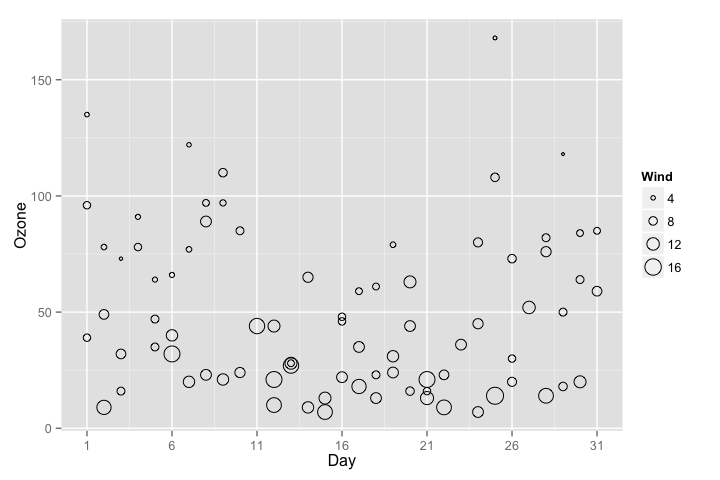To add a title, we include the option ggtitle and include the name of the graph as a string argument. To change the axis names we add x and y arguments to the labs command.

p6 <- p6 + ggtitle("Air Quality in New York by Day") +
labs(x = "Day of the month", y = "Ozone (ppb)")
p6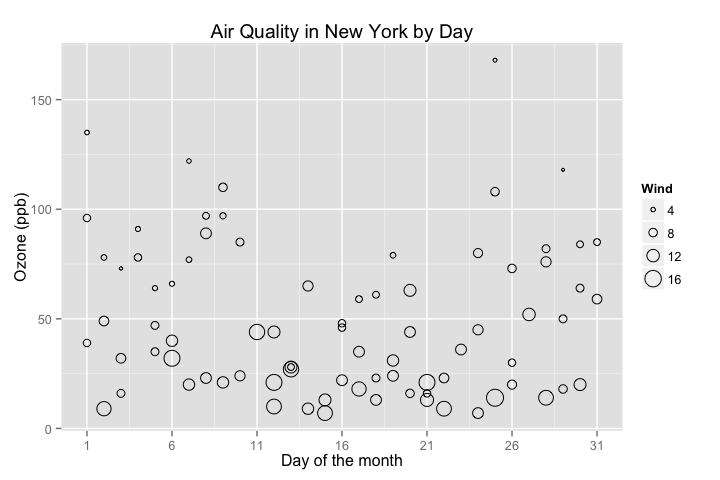There are a few options for adjusting the colour. The most simple is to make every point one fixed colour. You can reference colours by name, with the full list of colours recognised by R here. Let’s try making the outline mediumvioletred and the fill springgreen.

p6 <- ggplot(aq_trim, aes(x = Day, y = Ozone, size = Wind)) +
geom_point(shape = 21, colour = "mediumvioletred",
fill = "springgreen") +
ggtitle("Air Quality in New York by Day") +
labs(x = "Day of the month", y = "Ozone (ppb)") +
scale_x_continuous(breaks = seq(1, 31, 5))
p6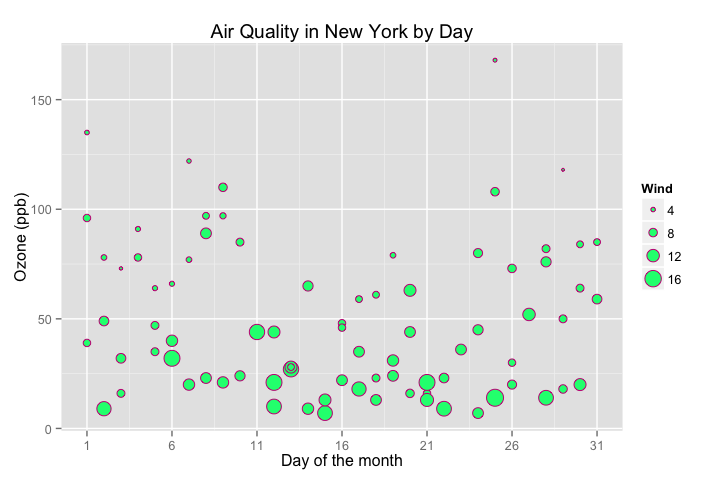You can change the colours using specific HEX codes instead. Here we have made the outline #000000 (black) and the fill “#40b8d0 (vivid cyan).

p6 <- ggplot(aq_trim, aes(x = Day, y = Ozone, size = Wind)) +
geom_point(shape = 21, colour = "#000000", fill = "#40b8d0") +
ggtitle("Air Quality in New York by Day") +
labs(x = "Day of the month", y = "Ozone (ppb)") +
scale_x_continuous(breaks = seq(1, 31, 5))
p6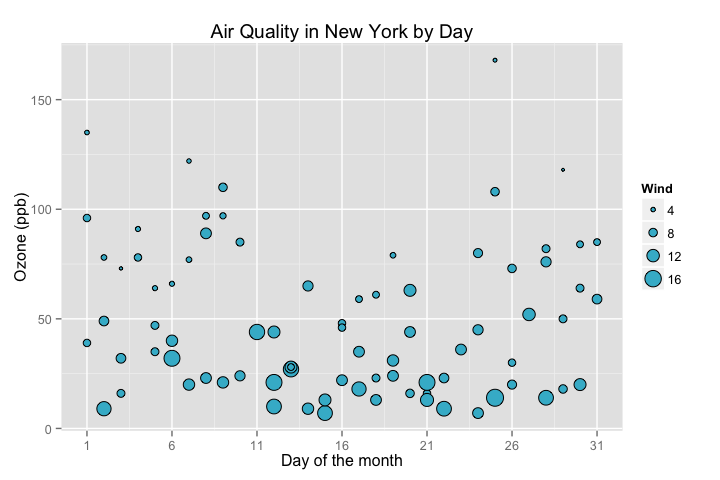You can also change the colour of the data points according to the levels of another variable. This can be done either as a continuous gradient, or as a levels of a factor variable. Let’s change the colour by the values of temperature:

p6 <- ggplot(aq_trim, aes(x = Day, y = Ozone, size = Wind, fill = Temp)) +
geom_point(shape = 21) +
ggtitle("Air Quality in New York by Day") +
labs(x = "Day of the month", y = "Ozone (ppb)") +
scale_x_continuous(breaks = seq(1, 31, 5))
p6We can change the gradient’s colours by adding the scale_fill_continuous option. The low and high arguments specify the range of colours the gradient should transition between.

p6 <-  p6 + scale_fill_continuous(low = "plum1", high = "purple4")
p6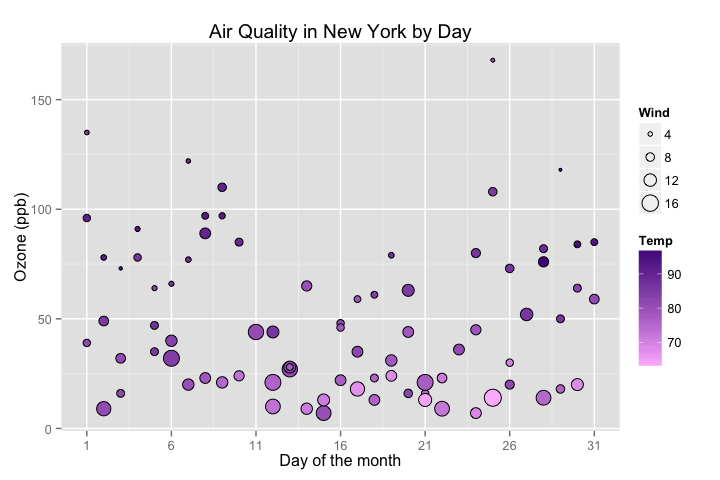We can see that higher temperatures seem to have higher ozone levels.

Let’s now change the colours of the data points by a factor variable, Month.

p6 <- ggplot(aq_trim, aes(x = Day, y = Ozone, size = Wind, fill = Month)) +
geom_point(shape = 21) +
ggtitle("Air Quality in New York by Day") +
labs(x = "Day of the month", y = "Ozone (ppb)") +
scale_x_continuous(breaks = seq(1, 31, 5))
p6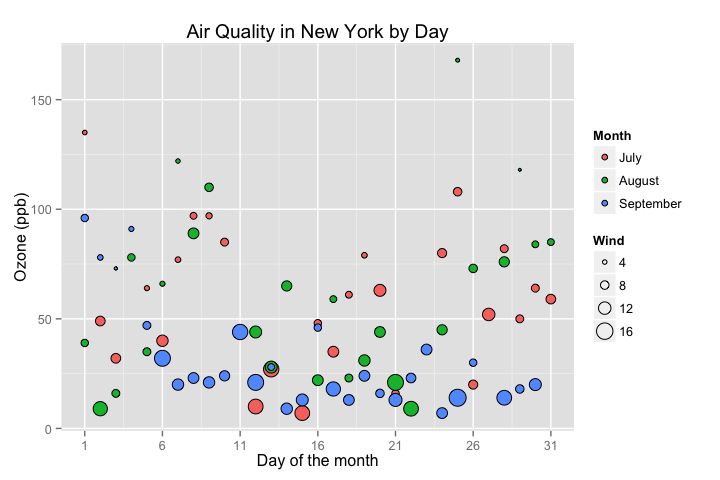Again, we can change the colours of these data points, this time using scale_fill_manual.

fill = c("steelblue", "yellowgreen", "violetred1")

p6 <- p6 + scale_fill_manual(values = fill)
p6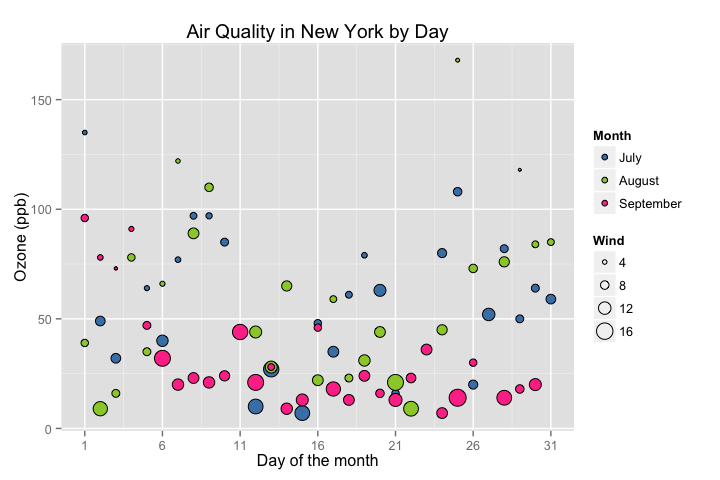### Adjusting the size of the data points

The default size of the the data points in a weighted scatterplot is mapped to the radius of the plots. If we want the data points to be proportional to the value of the weighting variable (e.g., a wind speed of 0 mph would have a value of 0), we need to use the scale_size_area.

p6 <- p6 + scale_size_area(max_size = 10)
p6For our graph, this makes the pattern for Wind a little hard to see. Another way to adjust the size of the data points is to use scale_size and specify a desired range.

p6 <- ggplot(aq_trim, aes(x = Day, y = Ozone, size = Wind, fill = Month)) +
geom_point(shape = 21) +
ggtitle("Air Quality in New York by Day") +
labs(x = "Day of the month", y = "Ozone (ppb)") +
scale_x_continuous(breaks = seq(1, 31, 5)) +
scale_fill_manual(values = fill) +
scale_size(range = c(1, 10))
p6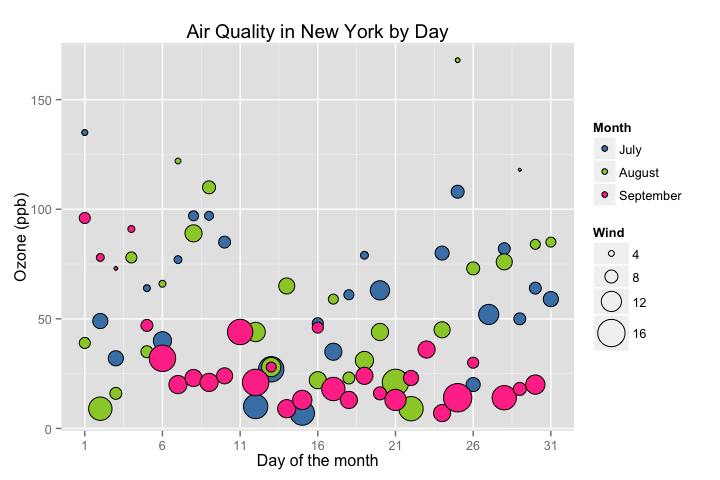To adjust the position of the legend from the default spot of right of the graph, we add the theme option and specify the legend.position = "bottom" argument. We can also change the legend shape using the legend.direction = "horizontal" argument.

p6 <- p6 + theme(legend.position = "bottom", legend.direction = "horizontal")
p6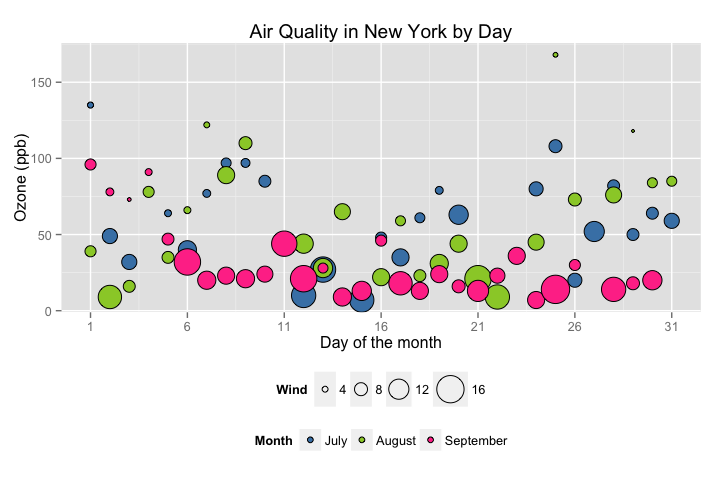### Changing the legend titles

To change the titles of the two legends, we use the labs option. In order to tell ggplot2 exactly what legend you’re referring to, just have a look in the ggplot option and see what argument you used to create the legend in the first place. In this case we used the size argument for “Wind” and fill for “Month”, so we pass these to labs with our new titles.

p6 <- p6 + labs(size = "Wind Speed (mph)", fill = "Months")
p6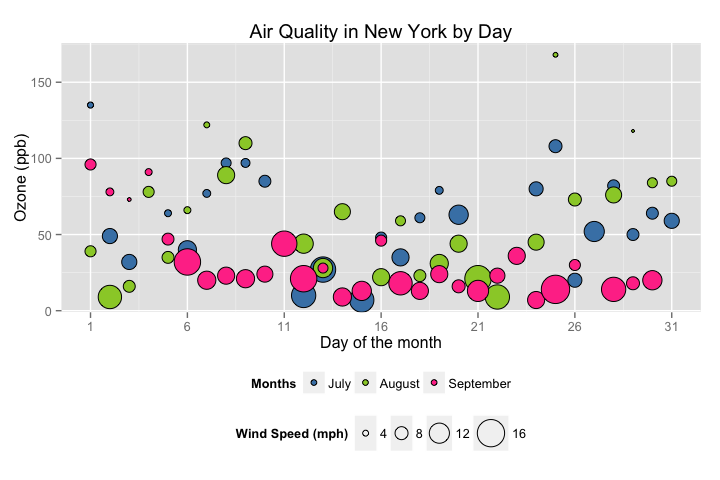### Creating horizontal legends

It looks a little awkward having the two titles sitting on top of each other, as well as taking up unnecessary space. To place the legends next to each other, we use the legend.box = "horizontal" argument in theme. Because the boxes around the legend keys aren’t even in each of the legends, this means the legends don’t align properly. To fix this, we change the box size around the legend keys using legend.key.size. We need to load in the grid package to get this argument to work.

library(grid)
p6 <- p6 + theme(legend.box = "horizontal",
legend.key.size = unit(1, "cm"))
p6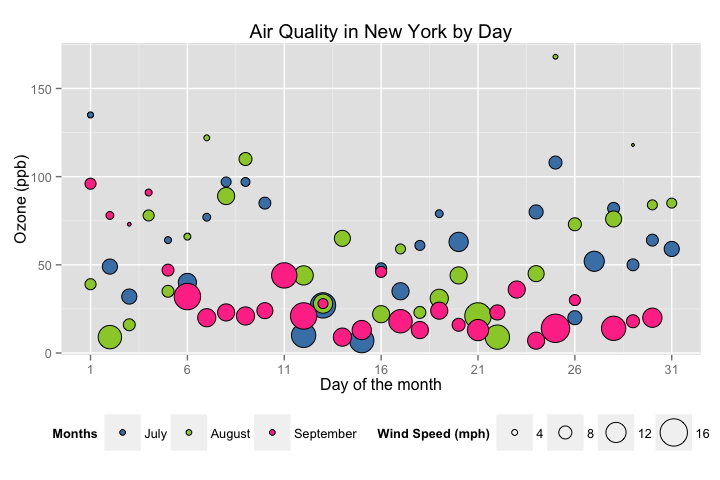### Using the white theme

As explained in the previous posts, we can also change the overall look of the plot using themes. We’ll start using a simple theme customisation by adding theme_bw() after ggplot(). As you can see, we can further tweak the graph using the theme option, which we’ve used so far to change the legend.

p6 <- ggplot(aq_trim, aes(x = Day, y = Ozone, size = Wind, fill = Month)) +
geom_point(shape = 21) +
theme_bw() +
theme() +
ggtitle("Air Quality in New York by Day") +
labs(x = "Day of the month", y = "Ozone (ppb)",
size = "Wind Speed (mph)", fill = "Months") +
scale_x_continuous(breaks = seq(1, 31, 5)) +
scale_fill_manual(values = fill) +
scale_size(range = c(1, 10)) +
theme(legend.position="bottom", legend.direction="horizontal",
legend.box = "horizontal",
legend.key.size = unit(1, "cm"))
p6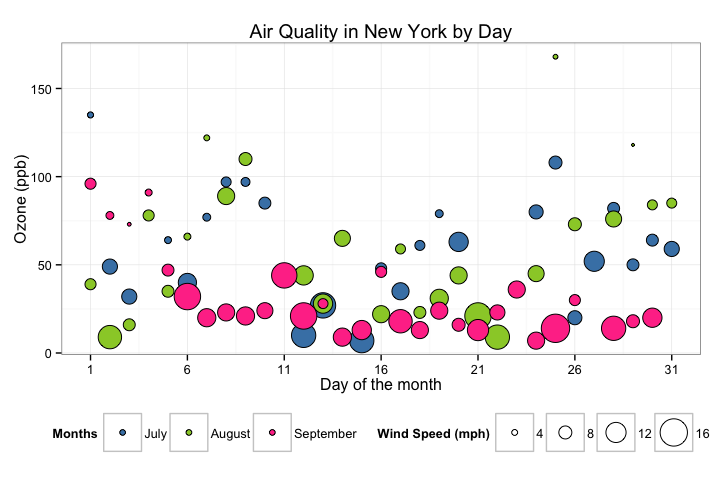### Creating an XKCD style chart

Of course, you may want to create your own themes as well. ggplot2 allows for a very high degree of customisation, including allowing you to use imported fonts. Below is an example of a theme Mauricio was able to create which mimics the visual style of XKCD. In order to create this chart, you first need to import the XKCD font, install it on your machine and load it into R using the extrafont package. These instructions are taken from here:

library(extrafont)

dest="xkcd.ttf", mode="wb")
system("mkdir ~/.fonts")
system("cp xkcd.ttf  ~/.fonts")
font_import(paths = "~/.fonts", pattern="[X/x]kcd")
fonts()


You can then create your graph:

fill <- c("#56B4E9", "#F0E442", "violetred1")

p6 <- ggplot(aq_trim, aes(x = Day, y = Ozone, size = Wind, fill = Month)) +
geom_point(shape = 21) +
ggtitle("Air Quality in New York by Day") +
labs(x = "Day of the month", y = "Ozone (ppb)",
size = "Wind Speed (mph)", fill = "Months") +
scale_x_continuous(breaks = seq(1, 31, 5)) +
scale_fill_manual(values = fill) +
scale_size(range = c(1, 10)) +
theme(legend.position="bottom", legend.direction="horizontal",
legend.box = "horizontal",
legend.key.size = unit(1, "cm"),
axis.line = element_line(size=1, colour = "black"),
panel.grid.major = element_blank(),
panel.grid.minor = element_blank(),
panel.border = element_blank(),
panel.background = element_blank(),
plot.title=element_text(family="xkcd-Regular"),
text=element_text(family="xkcd-Regular"),
axis.text.x=element_text(colour="black", size = 10),
axis.text.y=element_text(colour="black", size = 10))
p6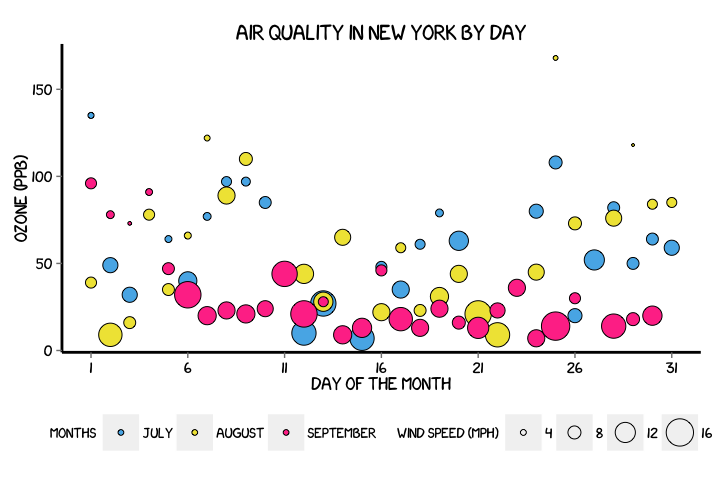### Using ‘The Economist’ theme

There are a wider range of pre-built themes available as part of the ggthemes package (more information on these here). Below we’ve applied theme_economist(), which approximates graphs in the Economist magazine.

library(ggthemes)

p6 <- ggplot(aq_trim, aes(x = Day, y = Ozone, size = Wind, fill = Month)) +
theme_economist() +
scale_fill_economist() +
geom_point(shape = 21) +
ggtitle("Air Quality in New York by Day") +
labs(x = "Day of the month", y = "Ozone (ppb)",
size = "Wind Speed (mph)", fill = "Months") +
scale_x_continuous(breaks = seq(1, 31, 5)) +
scale_size(range = c(1, 10)) +
theme(legend.position = "bottom", legend.direction = "horizontal",
legend.box = "horizontal",
legend.key.size = unit(1, "cm"),
plot.title = element_text(family="Tahoma"),
text = element_text(family = "Tahoma"),
axis.title = element_text(size = 12),
legend.text = element_text(size = 9),
legend.title=element_text(face = "bold", size = 9))
p6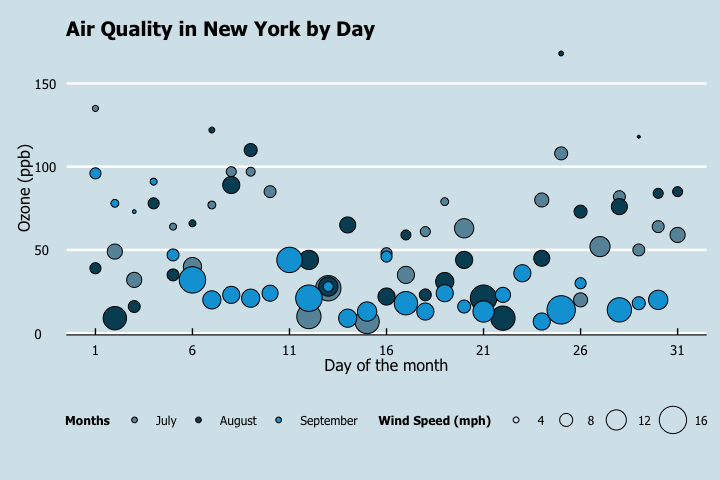As before, you can modify your plots a lot as ggplot2 allows many customisations. Here we present our original result shown at the top of page.

fill = c("steelblue", "yellowgreen", "violetred1")

p6 <- ggplot(aq_trim, aes(x = Day, y = Ozone, size = Wind, fill = Month)) +
geom_point(shape = 21) +
ggtitle("Air Quality in New York by Day") +
labs(x = "Day of the month", y = "Ozone (ppb)",
size = "Wind Speed (mph)", fill = "Months") +
scale_x_continuous(breaks = seq(1, 31, 5)) +
scale_size(range = c(1, 10)) +
scale_fill_manual(values = fill) +
theme(legend.position = "bottom", legend.direction = "horizontal",
legend.box = "horizontal",
legend.key.size = unit(1, "cm"),
axis.line = element_line(size=1, colour = "black"),
panel.grid.major = element_line(colour = "#d3d3d3"),
panel.grid.minor = element_blank(),
panel.border = element_blank(), panel.background = element_blank(),
plot.title = element_text(size = 14, family = "Tahoma", face = "bold"),
text=element_text(family="Tahoma"),
axis.text.x=element_text(colour="black", size = 9),
axis.text.y=element_text(colour="black", size = 9))
p6